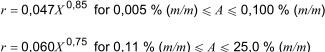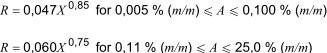ISO 3987 Petroleum products - Lubricating oils and additives - Determination of sulfated ash
10 Expression of results
Report the result to the nearest 0.001 % (m/m) for samples with sulfated ash contents below 0.02 % (m/m), and to the nearest 0.01 % (m/m) for those having higher levels.

11 Precision
The precision of this International Standard was established by statistical examination of interlaboratory results on test programmes that were run in 1975 and 1981.

Table 1 gives examples of specimen precision values using the formulae in 11.1 and 11.2.

11.1 Repeatability, r
The difference between two test results obtained by the same operator with the same apparatus under constant operating conditions on identical test material, would, in the long run, and in the normal and correct operation of the test method, exceed the following values in only one case in twenty:where X is the average of the results being compared, expressed as a percentage (mass fraction).

11.2 Reproducibility, R
The difference between two single and independent test results, obtained by different operators working in different laboratories on identical test material, would, in the long run, and in the normal and correct operation of the test method, exceed the following values in only one case in twenty:where X is the average of the results being compared, expressed as a percentage (mass fraction).

12 Test report
The test report shall contain at least the following information:
a) reference to this International Standard;
b) the type and complete identification of the product tested;
c) the result of the test (see Clause 10);
d) any deviation, by agreement or otherwise, from the procedure specified;
e) the date of the test.